#### Making good grids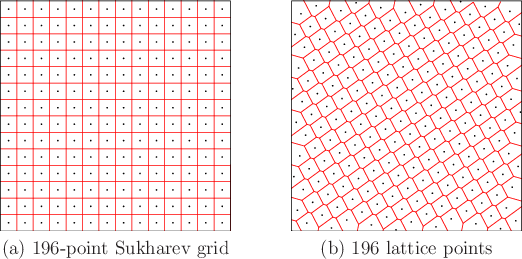Optimizing dispersion forces the points to be distributed more uniformly over. This causes them to fail statistical tests, but the point distribution is often better for motion planning purposes. Consider the best way to reduce dispersion if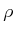is the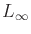metric and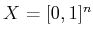. Suppose that the number of samples,, is given. Optimal dispersion is obtained by partitioning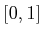into a grid of cubes and placing a point at the center of each cube, as shown for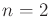and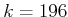in Figure 5.5a. The number of cubes per axis must be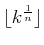, in which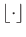denotes the floor. If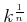is not an integer, then there are leftover points that may be placed anywhere without affecting the dispersion. Notice thatjust gives the number of points per axis for a grid ofpoints indimensions. The resulting grid will be referred to as a Sukharev grid .

The dispersion obtained by the Sukharev grid is the best possible. Therefore, a useful lower bound can be given for any setofsamples :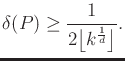(5.20)

This implies that keeping the dispersion fixed requires exponentially many points in the dimension,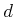.

At this point you might wonder whywas used instead of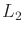, which seems more natural. This is because thecase is extremely difficult to optimize (except in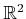, where a tiling of equilateral triangles can be made, with a point in the center of each one). Even the simple problem of determining the best way to distribute a fixed number of points in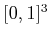is unsolved for most values of. See  for extensive treatment of this problem.

Suppose now that other topologies are considered instead of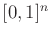. Let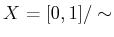, in which the identification produces a torus. The situation is quite different becauseno longer has a boundary. The Sukharev grid still produces optimal dispersion, but it can also be shifted without increasing the dispersion. In this case, a standard grid may also be used, which has the same number of points as the Sukharev grid but is translated to the origin. Thus, the first grid point is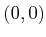, which is actually the same as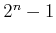other points by identification. Ifrepresents a cylinder and the number of points,, is given, then it is best to just use the Sukharev grid. It is possible, however, to shift each coordinate that behaves like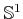. Ifis rectangular but not a square, a good grid can still be made by tiling the space with cubes. In some cases this will produce optimal dispersion. For complicated spaces such as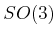, no grid exists in the sense defined so far. It is possible, however, to generate grids on the faces of an inscribed Platonic solid  and lift the samples to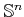with relatively little distortion . For example, to sample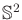, Sukharev grids can be placed on each face of a cube. These are lifted to obtain the warped grid shown in Figure 5.6a.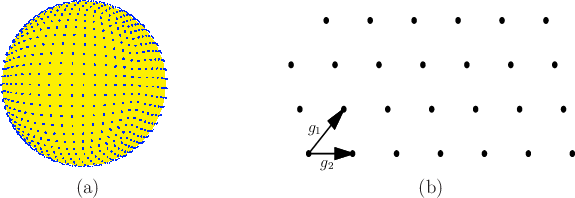Example 5..15 (Sukharev Grid)   Suppose thatand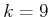. If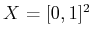, then the Sukharev grid yields points for the nine cases in which either coordinate may be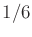,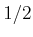, or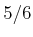. Thedispersion is. The spacing between the points along each axis is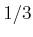, which is twice the dispersion. If instead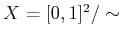, which represents a torus, then the nine points may be shifted to yield the standard grid. In this case each coordinate may be 0,, or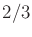. The dispersion and spacing between the points remain unchanged.One nice property of grids is that they have a lattice structure. This means that neighboring points can be obtained very easily be adding or subtracting vectors. Let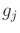be an-dimensional vector called a generator. A point on a lattice can be expressed as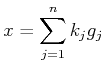(5.21)

forindependent generators, as depicted in Figure 5.6b. In a 2D grid, the generators represent up'' and right.'' If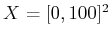and a standard grid with integer spacing is used, then the neighbors of the point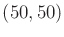are obtained by adding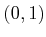,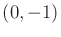,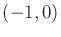, or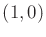. In a general lattice, the generators need not be orthogonal. An example is shown in Figure 5.5b. In Section 5.4.2, lattice structure will become important and convenient for defining the search graph.

Steven M LaValle 2020-08-14